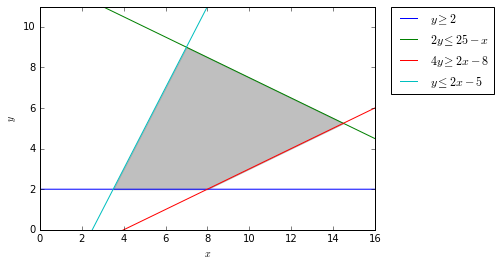## Linear Programming with Python and PuLP – Part 1

• Post author:
• Post category:Python

# Linear Programming with Python and PuLP – Part 1

## Introduction to Linear Programming

In this set of notebooks we will be looking at some linear programming problems and how we can construct and solve these problems using the python linear programming package PuLP.

We want to find the maximum solution to:

$Z = 4x + 3y$

This is known as our objective function. x and y in this equation are our decision variables.

In this example, the objective function is subject to the following constraints:

$x \geq 0 \\ y \geq 2 \\ 2y \leq 25 – x \\ 4y \geq 2x – 8 \\ y \leq 2x -5 \\$

We’ll begin by graphing this problem

In :
import numpy as np
import matplotlib.pyplot as plt
%matplotlib inline

# Construct lines
# x > 0
x = np.linspace(0, 20, 2000)
# y >= 2
y1 = (x*0) + 2
# 2y <= 25 - x
y2 = (25-x)/2.0
# 4y >= 2x - 8
y3 = (2*x-8)/4.0
# y <= 2x - 5
y4 = 2 * x -5

# Make plot
plt.plot(x, y1, label=r'$y\geq2') plt.plot(x, y2, label=r'$2y\leq25-x')
plt.plot(x, y3, label=r'$4y\geq 2x - 8') plt.plot(x, y4, label=r'$y\leq 2x-5')
plt.xlim((0, 16))
plt.ylim((0, 11))
plt.xlabel(r'$x) plt.ylabel(r'$y)
# Fill feasible region
y5 = np.minimum(y2, y4)
y6 = np.maximum(y1, y3)
plt.fill_between(x, y5, y6, where=y5>y6, color='grey', alpha=0.5)
plt.legend(bbox_to_anchor=(1.05, 1), loc=2, borderaxespad=0.)

Out:
<matplotlib.legend.Legend at 0x106a98410>Our solution lies somewhere in the grey feasible region in the graph above.

It has been proven that the minima and maxima of linear programming problems lie at the vertices of the feasible region. In this example, there are only 4 corners to our feasible region, so we can find the solutions for each corner to find our maximum.

The four corners are between the lines:

Line 1 Line 2
y ≥ 2 4y ≥ 2x – 8
2y ≤ 25 – x y ≤ 2x – 5
2y ≤ 25 – x 4y ≥ 2x – 8
y ≥ 2 y ≤ 2x – 5

So keeping in mind that:

$Z = 4x + 3y$

We can calculate Z for each corner:

1)

$y \geq 2 \text{ and } 4y \geq 2x – 8 \\ 2 = \dfrac{2x – 8}{4} \\ x = 8 \\ y = 2 \\ Z = (4 \times 8 + 3 \times 2) = 38 \\$

2)

$2y \leq 25 – x \text{ and } y \leq 2x – 5 \\ \dfrac{25 – x}{2} = 2x – 5 \\ x = 7 \\ y = 9 \\ Z = (4 \times 7 + 3 \times 9) = 55 \\$

3)

$2y \leq 25 – x \text{ and } 4y \geq 2x – 8 \\ \dfrac{25 – x}{2} = \dfrac{2x – 8}{4} \\ x = 14.5 \\ y = 5.25 \\ Z = (4 \times 14.5 + 3 \times 5.25) = 73.75 \\$

4)

$y \geq 2 \text{ and } y \leq 2x – 5 \\ 2 = 2x-5 \\ x = 3.5 \\ y = 2 \\ Z = (4 \times 3.5 + 3 \times 2) = 20$

We have successfully calculated that the maximum value for Z is 73.75, when x is 14.5 and y is 5.25.

This method of testing every vertex is only feasible for a small number of variables and constraints.
As the numbers of constraints and variables increase, it becomes far more difficult to graph these problems and work out all the vertices.
For example, if there were a third variable:

$Z = Ax + By + Cz$

We would have to graph in three dimensions (x, y and z).

In the next few notebooks, we’ll take a look at how we can use python and the PuLP package to solve this linear programming problem, as well as some more complex problems